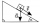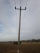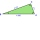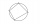# Angle + triangle - math problems

#### Number of problems found: 381

• Inclined planeThe body stays on an inclined plane and exerts a compressive force of 70N on it. Find the angle between the inclined plane and the horizontal if a gravitational force of 100N acts on the body.
• Diagonal BDFind the length of the diagonal BD in a rectangular trapezoid ABCD with a right angle at vertex A when/AD / = 8,1 cm and the angle DBA is 42°
• Telegraph polesThe bases of two adjacent telegraph poles have a height difference of 10.5 m. How long do the wires connect the two poles if the slope is 39° 30´?
• The cable carThe cable car is 2610 m long and rises at an angle of 35°. Calculate the height difference between the lower and upper station of the cable car.
• TV towerCalculate the height of the television tower if an observer standing 430 m from the base of the tower sees the peak at an altitude angle of 23°?Calculate the content of a regular 15-sides polygon inscribed in a circle with radius r = 4. Express the result to two decimal places.
• ParallelogramFind the perimeter of the parallelogram, where base a = 8 cm, height v = 3 cm, and angle alpha = 35° is the magnitude of the angle at vertex A.
• Triangular prismCalculate the surface of a regular triangular prism, the edges of the base are 6 cm long and the height of the prism is 15 cm.
• Sphere in coneA sphere is inscribed in the cone (the intersection of their boundaries consists of a circle and one point). The ratio of the surface of the ball and the contents of the base is 4: 3. A plane passing through the axis of a cone cuts the cone in an isoscele
• BisectorsAs shown, in △ ABC, ∠C = 90°, AD bisects ∠BAC, DE⊥AB to E, BE = 2, BC = 6. Find the perimeter of triangle △ BDE.
• Interior anglesCalculate the interior angles of a triangle that are in the ratio 2: 3: 4.
• Observation towerFrom the observation tower at a height of 105 m above sea level, the ship is aimed at a depth angle of 1° 49´. How far is the ship from the base of the tower?
• SailboatThe 20 m long sailboat has an 8 m high mast in the middle of the deck. The top of the mast is fixed to the bow and stern with a steel cable. Determine how much cable is needed to secure the mast and what angle the cable will make with the ship's deck.
• Distance of pointsA regular quadrilateral pyramid ABCDV is given, in which edge AB = a = 4 cm and height v = 8 cm. Let S be the center of the CV. Find the distance of points A and S.
• Powerplant chimneyFrom the window of the building at a height of 7.5 m, the top of the factory chimney can be seen at an altitude angle of 76° 30 ′. The base of the chimney can be seen from the same place at a depth angle of 5° 50 ′. How tall is the chimney?
• The rescue helicopterThe rescue helicopter is above the landing site at a height of 180m. The site of the rescue operation can be seen from here at a depth angle of 52° 40 '. How far will the helicopter land from the rescue site?
• Clock handsCalculate the internal angles of a triangle whose vertices lie on the clock's 2, 6 and 11 hours.
• Interior anglesIn a quadrilateral ABCD, whose vertices lie on some circle, the angle at vertex A is 58 degrees and the angle at vertex B is 134 degrees. Calculate the sizes of the remaining interior angles.
• The angle of viewDetermine the angle of view at which the observer sees a rod 16 m long when it is 18 m from one end and 27 m from the other.
• Two chordsFrom the point on the circle with a diameter of 8 cm, two identical chords are led, which form an angle of 60°. Calculate the length of these chords.

Do you have an interesting mathematical word problem that you can't solve it? Submit a math problem, and we can try to solve it.

We will send a solution to your e-mail address. Solved examples are also published here. Please enter the e-mail correctly and check whether you don't have a full mailbox.

Please do not submit problems from current active competitions such as Mathematical Olympiad, correspondence seminars etc...